Next: Coordinate Transformations Up: Vector Algebra and Vector Previous: Vector Algebra

Cartesian Components of a Vector

Consider a Cartesian coordinate system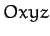consisting of an origin,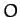, and three mutually perpendicular coordinate axes,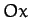,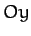, and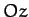--see Figure A.99. Such a system is said to be right-handed if, when looking along thedirection, a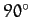clockwise rotation aboutis required to takeinto. Otherwise, it is said to be left-handed. In physics, it is conventional to always use right-handed coordinate systems.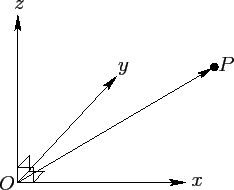It is convenient to define unit vectors,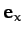,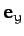, and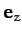, parallel to,, and, respectively. Incidentally, a unit vector is a vector whose magnitude is unity. The position vector,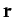, of some general pointwhose Cartesian coordinates are (,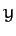,) is then given by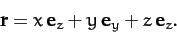(1271)

In other words, we can get fromtoby moving a distanceparallel to, then a distanceparallel to, and then a distanceparallel to. Similarly, if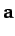is an arbitrary vector then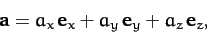(1272)

where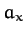,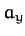, and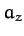are termed the Cartesian components of. It is coventional to write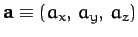. It follows that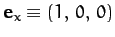,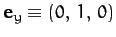, and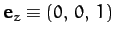. Of course,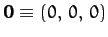.

According to the three-dimensional generalization of the Pythagorean theorem, the distance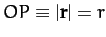is given by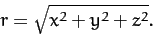(1273)

By analogy, the magnitude of a general vectortakes the form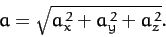(1274)

Ifand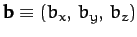then it is easily demonstrated that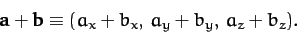(1275)

Furthermore, ifis a scalar then it is apparent that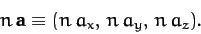(1276)Next: Coordinate Transformations Up: Vector Algebra and Vector Previous: Vector Algebra
Richard Fitzpatrick 2011-03-31